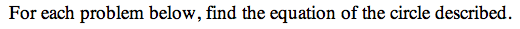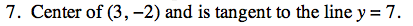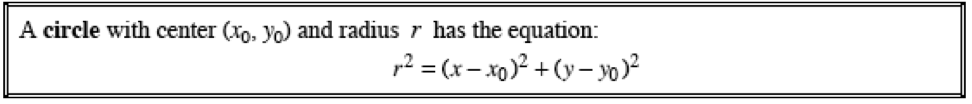13C-7.

### Missing problem for Book PCT => Chapter Ch13 => Lesson 13.1.1 => Problem 13C-7

Created from orphaned homework help problemIs the tangent line a horizontal or vertical line? Draw a perpendicular line from the center of the circle to the tangent line. What is the distance between the center and this new point?